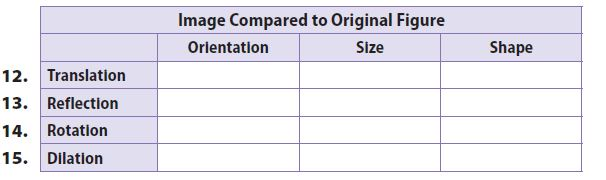Homework Explained - Math Practice 101Dear guest, you are not a registered member. As a guest, you only have read-only access to our books, tests and other practice materials.

As a registered member you can:

Registration is free and doesn't require any type of payment information. Click here to Register.
Go to page:
Chapter 10:Transformations and Similarity; Lesson 1: Properties of Dilations

### 10.1 Independent Practice

For 7–11, tell whether one figure is a dilation of the other or not. Explain your reasoning.

• Question 7

Quadrilateral MNPQ has side lengths of 15 mm, 24 mm, 21 mm, and 18 mm. Quadrilateral M′N′P′Q′ has side lengths of 5 mm, 8 mm, 7 mm, and 4 mm.

• Question 8

Triangle RST has angles measuring 38° and 75°. Triangle R′S′T ′ has angles measuring 67° and 38°. The sides are proportional.

• Question 9

Two triangles, Triangle 1 and Triangle 2, are similar.

• Question 10

Quadrilateral MNPQ is the same shape but a different size than quadrilateral M′N′P′Q.

• Question 11

On a coordinate plane, triangle UVW has coordinates U(20, −12), V(8, 6), and W(−24, -4). Triangle U′V′W′ has coordinates U′(15, −9), V′(6, 4.5), and W′(−18, -3).

Complete the table by writing “same” or “changed” to compare the image with the original figure in the given transformation.

• Question 12• Type below:
• Question 16

Describe the image of a dilation with a scale factor of 1.

Yes, email page to my online tutor. (if you didn't add a tutor yet, you can add one here)